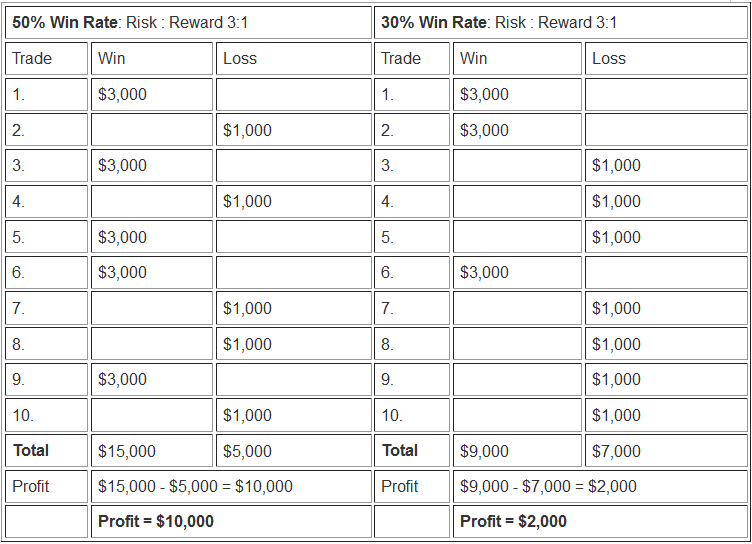# Money Management Methods - Indices Money Management Strategies

In stock index there are two money management methods commonly used to manage the account capital in a traders account.

The two money management methods are:

1. Risk: Reward Ratio

2. Percentage Risk Method

## Risk: Reward Ratio

The risk: reward ratios means that as trader you only trade when you are likely to make more profits, for example if you have a risk: reward ratio of 3:1 then it means that you will only trade when you are likely to make profits 3 times as compared to not making profits.

Or put in another way you are more likely to make 3 times what you risk in a single trade. This means that if you risk \$100 then you are likely to make \$300 dollar in profit on that trade.

Having a high risk: reward ratio will improve your chances of making more profit when trading the stock index market, for example even if your system win rate is 50 % or even 30 %, you will still make profits if you have a good risk: reward ratio as shown in the illustration below:## Percentage Risk Method

This method a trader will set a fixed percent to risk per trade, therefore a trader may decide to risk only 2 % per trade, this way their risk is always kept to a minimum.

Therefore if a trader has a \$50,000 dollar account, the amount to risk per trade is about \$1,000 dollars, therefore depending on the trade transaction open a trader will set the stop at this amount of \$1,000 dollars:

### For example:

If the trader opens is trading the Italy FTSE MIB 40 or IT40Cash which has a pip value of € 1 and one pip move is equal to 1 point then the trader would calculate the percent risk money management depending on the trading lots opened as shown below

Example 1:

If the trader opens 1 Lot and 1 point move is equal to € 1 which is equal to \$1.2, then the trader would calculate the points where to place a stop on their trade as shown below:

1 Lot equals € 1 per 1 pip move (\$1.2)

\$1,000 dollars/\$1.2 (€ 1 is equal to \$1.2) equals 833 points

The trader would set their stop 833 pips away

Example 2:

3 Lots equals € 3 per 1 pip move (\$3.6)

If the trader opens 3 Lot and 1 point move is equal to € 3 which is equal to \$3.6, then the trader would calculate the points where to place a stop on their trade as shown below:

\$1,000 dollars/\$3.6 (€ 3 is equal to \$3.6) equals 277 points

The trader would set their stop 277 pips away

Example 3:

5 Lots equals € 5 per 1 pip move (\$6)

If the trader opens 5 Lots and 1 point move is equal to € 5 which is equal to \$6, then the trader would calculate the points where to place a stop on their trade as shown below:

\$1,000 dollars/\$6 (€ 5 is equal to \$6) equals 166 points

The trader would set their stop 166 pips away

Example 4:

10 Lots equals € 10 per 1 pip move (\$12)

If the trader opens 10 Lots and 1 point move is equal to € 10 which is equal to \$12, then the trader would calculate the points where to place a stop on their trade as shown below:

\$1,000 dollars/\$12 (€ 10 is equal to \$12) equals 83 points

The trader would set their stop 83 pips away

This also means the less the lots a trader opens, the more the pips a trader has when it comes to setting the stops. If a trader opens 1 lot then he can set the stop up to 833 pips and still be within the 2 % percent risk rule, while if the trader opens 10 lots then they will have to set the stops at only 83 points away so as to be within the 2 % risk management rule.